Question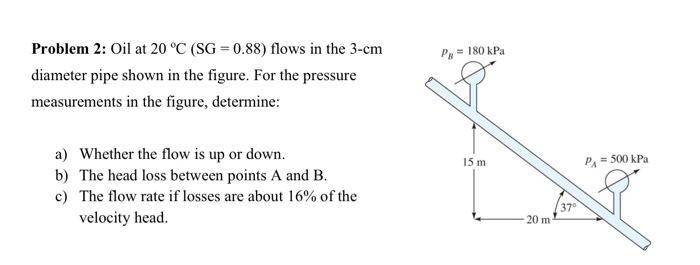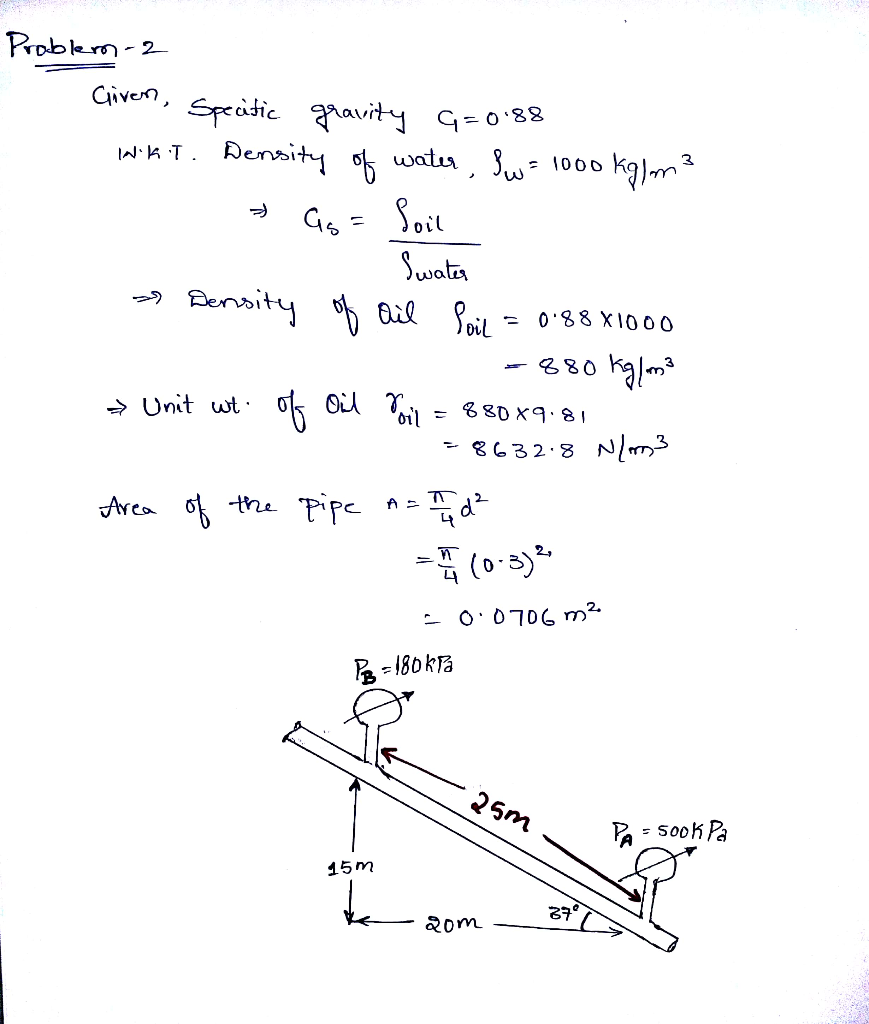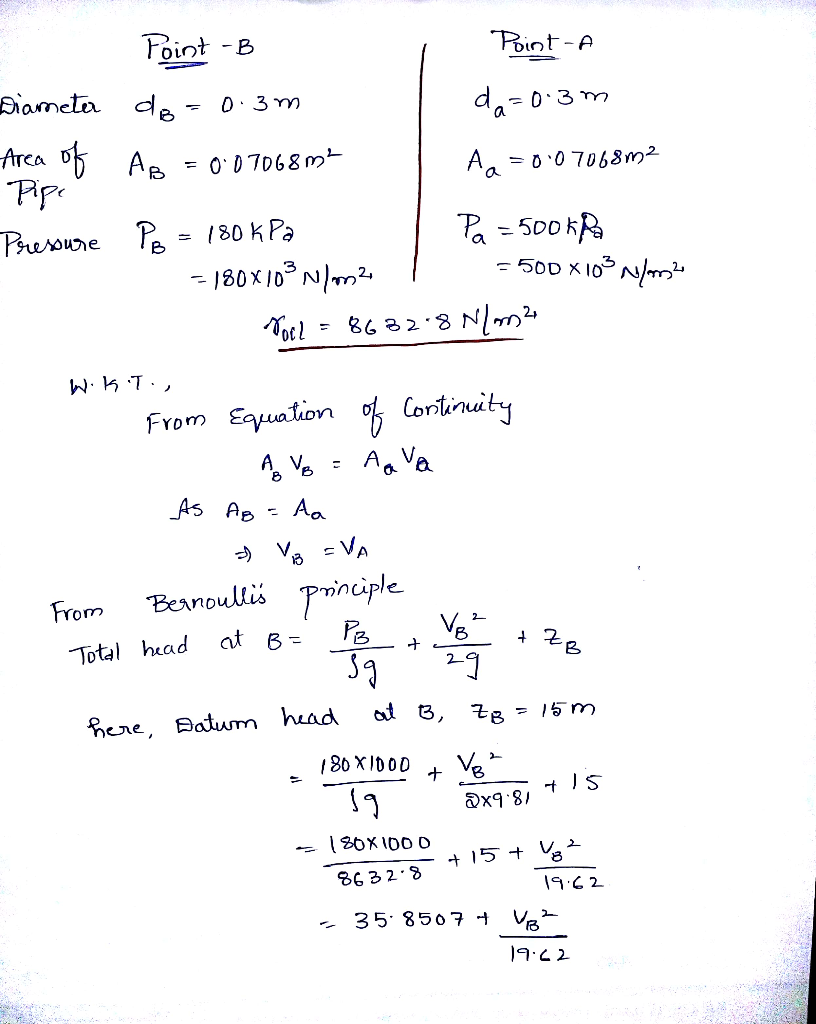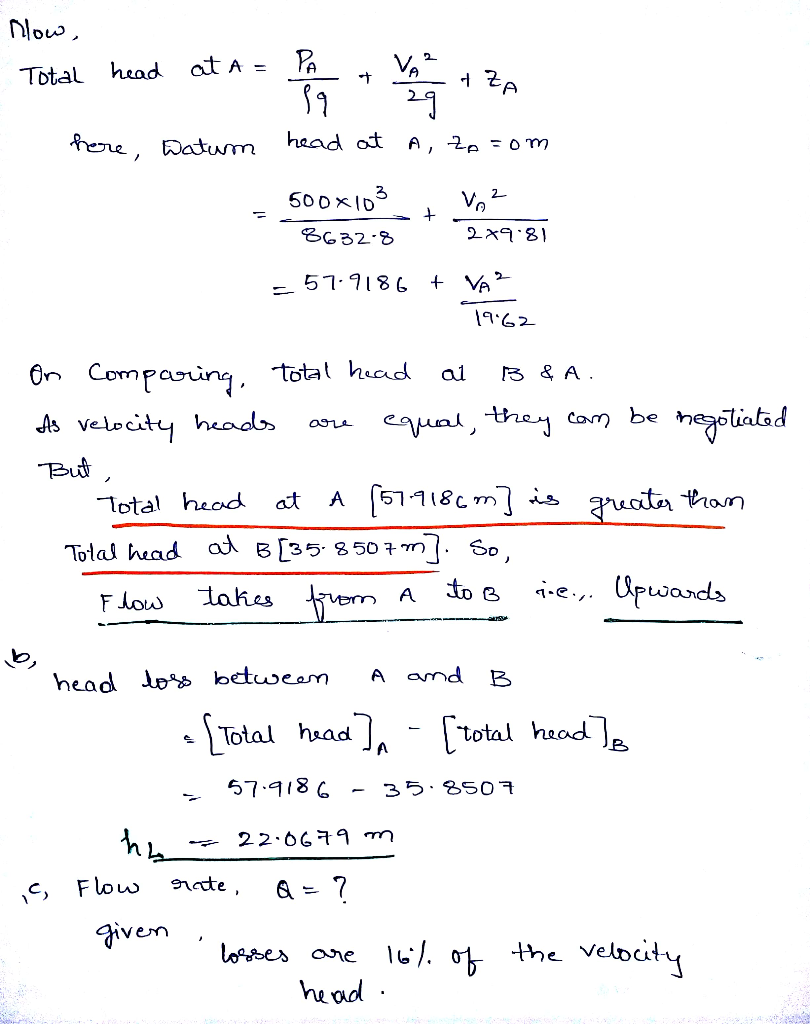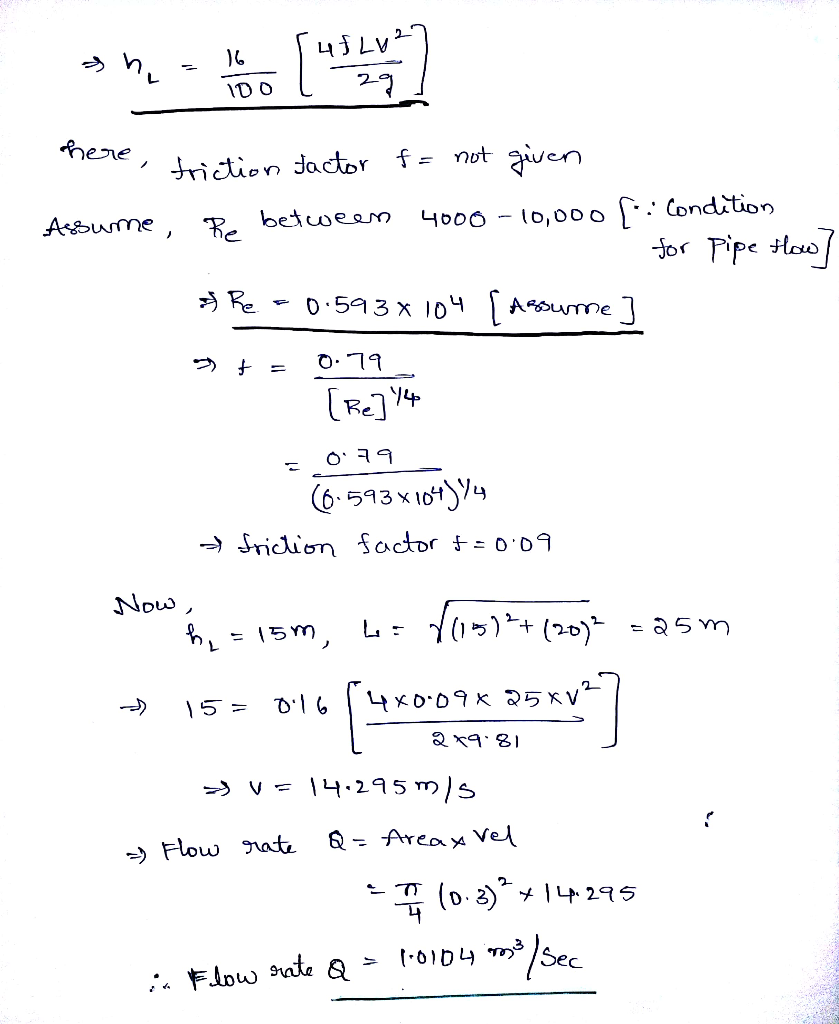#### Earn Coins

Coins can be redeemed for fabulous gifts.

Similar Homework Help Questions
• ### A flow nozzle equipped with a differential pressure gage is used to measure the flow rate of water at 10°C (p 9997 kg/m3 and p 1.307 x 10-3 kg/m s) through a 3-cm-diameter horizontal pipe. The nozzle...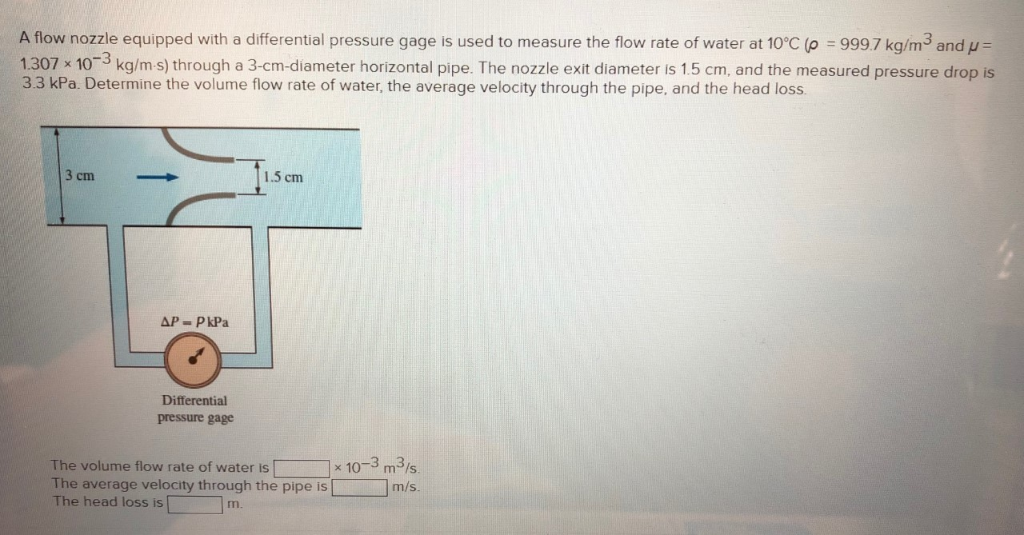A flow nozzle equipped with a differential pressure gage is used to measure the flow rate of water at 10°C (p 9997 kg/m3 and p 1.307 x 10-3 kg/m s) through a 3-cm-diameter horizontal pipe. The nozzle exit diameter is 1.5 cm, and the measured pressure drop is 3.3 kPa. Determine the volume flow rate of water, the average velocity through the pipe, and the head loss 1.5 cm Differential pressure gage -3 m3(s m/s The volume flow rate of...

• ### Glycerin at 20 °C flows upward in a vertical 75-mm-diameter pipe with a centerline velocity of...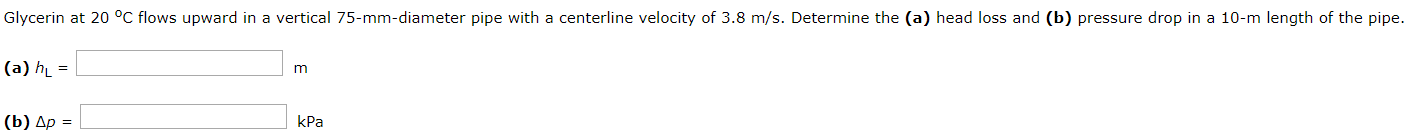Glycerin at 20 °C flows upward in a vertical 75-mm-diameter pipe with a centerline velocity of 3.8 m/s. Determine the (a) head loss and (b) pressure drop in a 10-m length of the pipe. (a) h kPa (b) Др —

• ### (a) A horizontal pipe of diameter 10 cm carries crude oil from A to C as...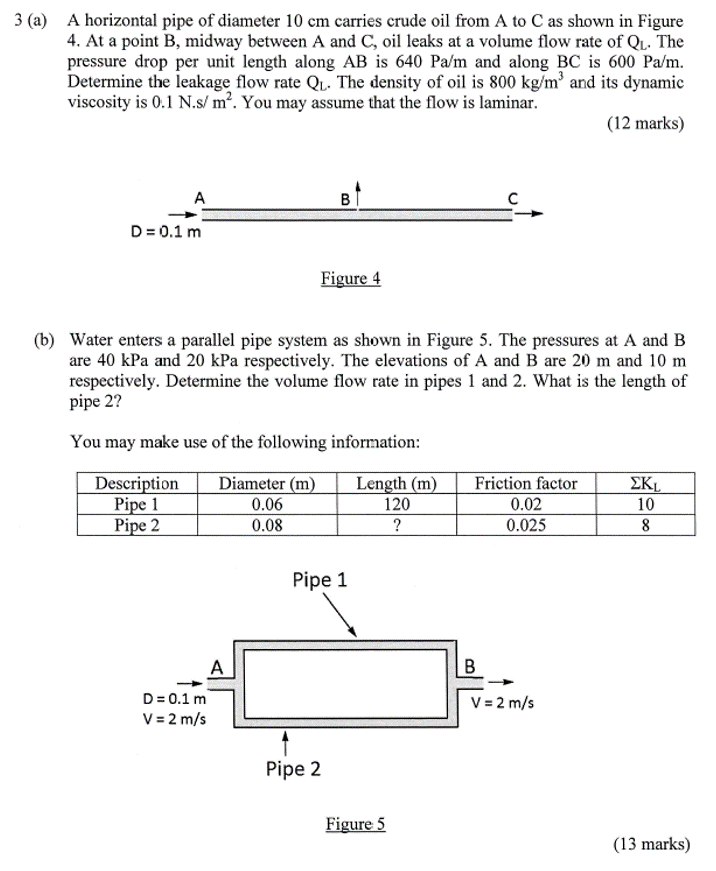(a) A horizontal pipe of diameter 10 cm carries crude oil from A to C as shown in Figure 4. At a point B, midway between A and C, oil leaks at a volume flow rate of QL . The pressure drop per unit length along AB is 640 Pa/m and along BC is 600 Pa/m. Determine the leakage flow rate QL . The density of oil is 800 kg/m3 and its dynamic viscosity is 0.1 N.s/ m2 . You...

• ### Problem 3: The following data were obtained for flow of 20°C water at 20 m/hr through a badly corroded 5-cm-diameter pipe which slopes downward at an angle of 8°: pı -420 kPa, zi -12 m, p2- 250 kPa,...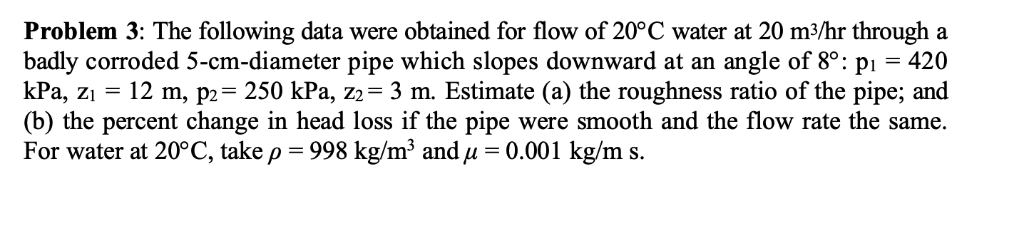Problem 3: The following data were obtained for flow of 20°C water at 20 m/hr through a badly corroded 5-cm-diameter pipe which slopes downward at an angle of 8°: pı -420 kPa, zi -12 m, p2- 250 kPa, z2- 3 m. Estimate (a) the roughness ratio of the pipe; and (b) the percent change in head loss if the pipe were smooth and the flow rate the same. For water at 20°C, take ρ-998 kg/m3 and μ-0.001 kg/m s Problem...

• ### 6-7. Oil flows through the 100-mm-diameter pipe with a velocity of 8 m/s. If the pressure...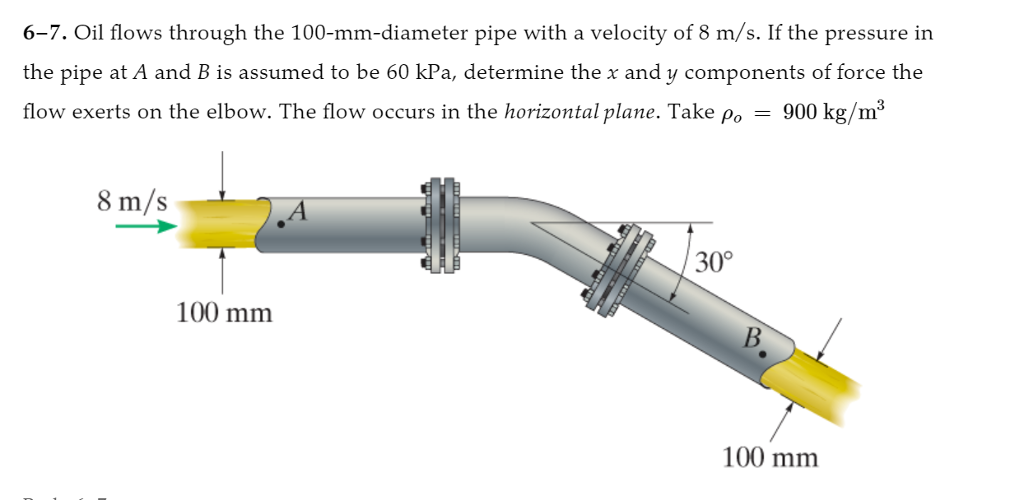6-7. Oil flows through the 100-mm-diameter pipe with a velocity of 8 m/s. If the pressure in the pipe at A and B is assumed to be 60 kPa, determine the x and y components of force the flow exerts on the elbow. The flow occurs in the horizontal plane. Take ρο -900 kg/m3 8 m/s 30° 100 mm 100 mm

• ### -2 2 Pipe flow [6 points) A liquid with SG 0.96, 1 = 9.2 x 10-4...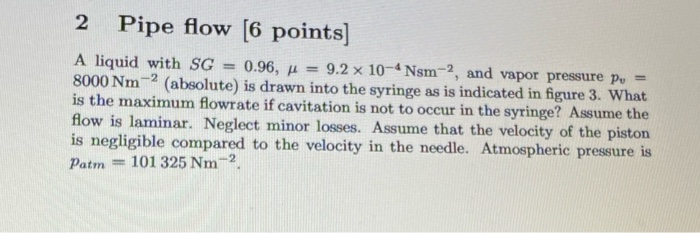-2 2 Pipe flow [6 points) A liquid with SG 0.96, 1 = 9.2 x 10-4 Nsm-2, and vapor pressure pu = 8000 Nm (absolute) is drawn into the syringe as is indicated in figure 3. What is the maximum flowrate if cavitation is not to occur in the syringe? Assume the flow is laminar. Neglect minor losses. Assume that the velocity of the piston is negligible compared to the velocity in the needle. Atmospheric pressure is Patm 101 325...

• ### Problem 2 Let's consider a single-stream problem with a 28-cm diameter pipe where Refrigerant R-134a flows...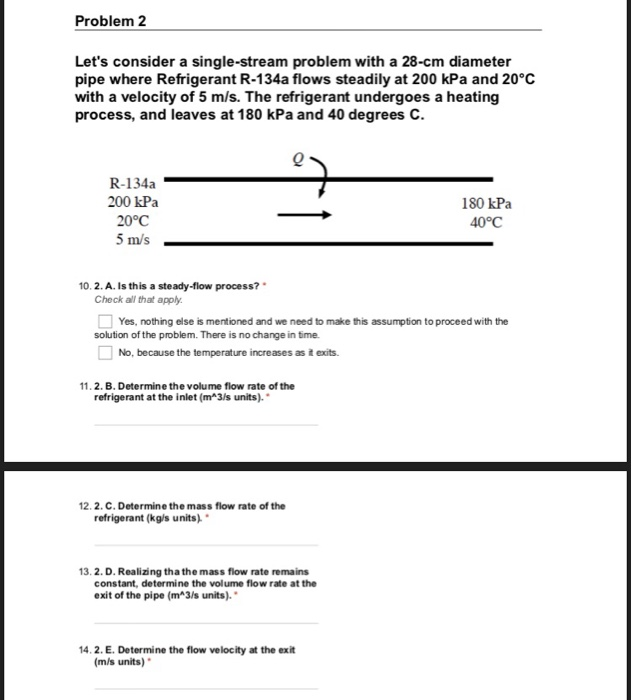Problem 2 Let's consider a single-stream problem with a 28-cm diameter pipe where Refrigerant R-134a flows steadily at 200 kPa and 20°C with a velocity of 5 m/s. The refrigerant undergoes a heating process, and leaves at 180 kPa and 40 degrees C 0 R-134a 200 kPa 20°C 5 m/s 180 kPa 40°C 10.2. A. Is this a steady-flow process? Check all that apply. □ Yes, nothing else is mentioned and we need to make this assumption to proceed with...

• ### Water is delivered to a 4.0 cm diameter fountain head from a 6.9 cm diameter pipe...

Water is delivered to a 4.0 cm diameter fountain head from a 6.9 cm diameter pipe that is 3.4 m below the fountain head and at a pressure of 94 kPa. The fountain head shoots the water straight up into the air. Neglect all losses. a.) What is the velocity of water coming out of the fountain head? Hint: Must use Bernoulli AND Continuity b.) What is the volume flow rate of the water through the fountain head? Hint: Q=vA

• ### Chapters Six & Seven Problems: 6.11 The venture meter shown in Figure 6.4 carries oil (sg-0.85)....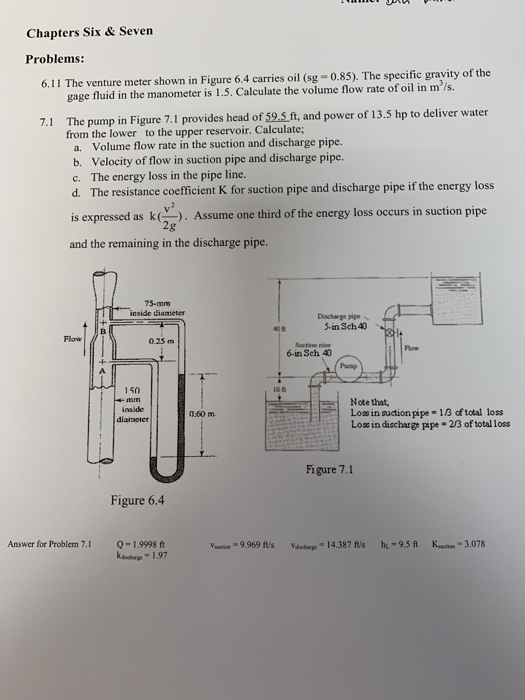Chapters Six & Seven Problems: 6.11 The venture meter shown in Figure 6.4 carries oil (sg-0.85). The specific gravity of the gage fluid in the manometer is 1.5. Calculate the volume flow rate of oil in m'/s. 7.1 The pump in Figure 7.1 provides head of 59.5 ft, and power of 13.5 hp to deliver water from the lower to the upper reservoir. Calculate; a. Volume flow rate in the suction and discharge pipe. b. Velocity of flow in suction...

• ### DON’T disregard the thermal conductivity of the pipe 3.) Oil at 200 C flows in a pipeline. The pipe has a 30-cm diameter and the flow is at an average velocity of 2 m/s. A 200-m-long section of t...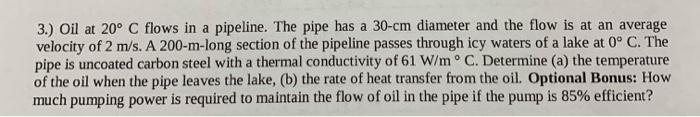DON’T disregard the thermal conductivity of the pipe 3.) Oil at 200 C flows in a pipeline. The pipe has a 30-cm diameter and the flow is at an average velocity of 2 m/s. A 200-m-long section of the pipeline passes through icy waters of a lake at 0° C. The pipe is uncoated carbon steel with a thermal conductivity of 61 W/m o C. Determine (a) the temperature of the oil when the pipe leaves the lake, (b) the...